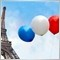# Help please to program maximum SL on EA2161

Hi everybody,

I come to you because I need a clarification. So I programmed an Expert advisor, he uses the ATR as SL. What I would like to be able to do is tell my expert advisor not to take into account the SL of the ATR if it is more than 200 pips for example.
If the ATR wants to place an SL at more than 200 pips, then our Expert Advisor will not take into account the SL of the ATR, but the one that will have defined by default, 200 pips.

This is the part of the code that interests us:

{
double SL=0, TP=0;
int  T=0;
bool OpenBar=true;
//---
if(EnterOpenBar) if(iVolume(NULL,0,0)>1) OpenBar=false;
//---
if (OpenBar)
{
//---
if(StopLoss>0)
else
if(StopLoss<=0)
SL=Bid-ATR_Multiplicator*iATR(Symbol(),0,ATR_Level,0);
//---
if(TakeProfit>0)
else
if(TakeProfit<=0)
TP=Bid+ATR_Multiplicator*iATR(Symbol(),0,ATR_Level,0);
{
//---
if (TotalOrders()<1
//---
&& (iMA(Symbol(),MA_Time_Frames,P3,0,MA_MODE,MA_Applied_Price,1) > iMA(Symbol(),MA_Time_Frames,P4,0,MA_MODE,MA_Applied_Price,1)))
//---
{
{
}
//---
{
}
//---
}
}
}
}
As can be seen, one has an SL that can be programmed with the number of pips we want, otherwise if the SL is at 0 in the parameters then the ATR calculates the SL.

But how do you explain to EA that you do not want to have a SL of more than 200 pips ?!

If SL of ATR > 200 pips them SL = 200 pips.

How do you code that?

If anyone could help me please. thank you.40785

Say the truth, we don't like liar here.2161

Alain Verleyen:

Say the truth, we don't like liar here.

Sorry if my English is not correct, but I use the google translator. So I'm not a "liar" as you can mean .. I programmed this EA by deciphering the expert advisor that I had done done by a programmer. With every job I've given to a programmer in the past, it has allowed me to learn the basics of mt4 programming. So that does not answer my problem, because I do not know how one can define a limit of SL to an EA; I can not find anything on the internet that can clarify.40785

Sebastien Pelle:
Sorry if my English is not correct, but I use the google translator. So I'm not a "liar" as you can mean .. I programmed this EA by deciphering the expert advisor that I had done done by a programmer. With every job I've given to a programmer in the past, it has allowed me to learn the basics of mt4 programming. So that does not answer my problem, because I do not know how one can define a limit of SL to an EA; I can not find anything on the internet that can clarify.
Then just continue to learn.40785

Sebastien Pelle:
I would like, but I can not find information on google related to this subject. If you have links to share about this kind of topic, I would be interested. If not for denigration, you can keep quiet.

I am very quiet, thanks.

You have it almost :

If SL of ATR > 200 pips them SL = 200 pips.

How would you code "SL of ATR" ?

How would you code "200 pips" ?2161

Alain Verleyen:
Then just continue to learn.
AH good link thank you. With this explanation I will be able to do what I want.2161

I first modified the use I made of the else

{
//---
if(StopLoss_R>0)
else
SL=Bid-ATR_Multiplicator_R*iATR(Symbol(),0,ATR_Level_R,0);
//---
if(TakeProfit_R>0)
else
TP=Bid+ATR_Multiplicator_R*iATR(Symbol(),0,ATR_Level_R,0);
{

Now I'm trying to figure out how to set the limit.2161

//---
if(StopLoss_R>0)
{
}
else if(StopLoss_R<=0)
{
SL=Bid-ATR_Multiplicator_R*iATR(Symbol(),0,ATR_Level_R,0);
}
else if(SL>=201)
{
}
//---
Could it be written like that?2161

Sebastien Pelle:
//---
if(StopLoss_R>0)
{